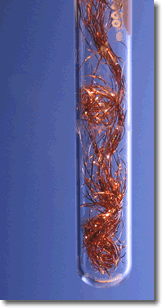# 16.1: Oxidation and Reduction- Some Definitions

"Redox" is short for "oxidation and reduction", two complimentary types of chemical reactions. The term oxidation originally referred to substances combining with oxygen, as happens when an iron bar rusts or a campfire log burns. We often refer to these two examples as corrosion and combustion. Reduction originally referred to the process of converting metal ores to pure metals, a process that is accompanied by a reduction in the mass of the ore.

These two terms have broader meanings now. In all oxidation-reduction reactions an exchange of electrons occurs - one substance loses electrons while something else gains them. That is the key to understanding redox reactions. We'll define these terms below.Figure $$\PageIndex{1}$$: Reaction of copper wire in a silver nitrate solution.

A simple demonstration of a redox reaction involves placing a solid piece of copper wire in a silver nitrate solution. Within minutes the wire begins to look fuzzy or furry, as small silver crystals begin to form on the wire. Meanwhile, the originally clear silver nitrate solution begins to take on a pale bluish tint. Furthermore, if the crystals are shaken off of the wire we see that the wire partially disintegrated.

The overall equation for our demonstration describes the events:

$\ce{Cu(s) + 2AgNO3(aq) → Cu(NO3)2 (aq) + 2 Ag(s)}$

Remember that when we have aqueous solutions of ionic compounds, the ions are really present as separate ions, not as bonded particles. So we can write an expanded equation:

$\ce{Cu(s) + 2Ag^{+}(aq) + 2NO^{-}3(aq) → Cu^{2+}(aq) + 2NO^{-}3(aq) + 2Ag(s)}$

Finally we remove spectator ions from the equation. Remember that spectator ions are ions that undergo no change during a reaction. Notice the nitrate ions, $$NO_3^-$$ – they start as aqueous ions and end up exactly the same.

Removing the spectator ions gives us our net ionic equation:

$\ce{Cu(s) + 2Ag^{+}(aq) → Cu^{2+}(aq) + 2Ag(s)}$

We can now see a bit more clearly what changes are occurring with this reaction.

## Oxidation of Copper Metal to Make Copper Ions

Copper began as a neutral atom with no charge, but changed into an ion with a charge of +2. An atom becomes a positive ion by losing electrons:

$\ce{Cu(s) → Cu^{2+}(aq) + 2e^{-}} \label{oxeq}$

Notice that copper began as a solid but is converted into aqueous ions - this is why the copper wire disintegrates. We say that copper was oxidized because is has lost electrons (i.e., electrons appear on the product side of the Equation $$\ref{oxeq}$$).

## Reduction of Silver Ions to Make Silver Metal

Silver was converted from an ion with a charge of +1, Ag+, to a neutral atom, Ag. The only way an ion can undergo this change is to gain an electron:

$\ce{Ag^{+}(aq) + e^{-} → Ag(s)} \label{redeq}$

Notice that solid silver is formed - this is what causes the fuzzy appearance to begin appearing on the wire - solid silver crystals. Silver has gained electrons - it has been reduced (i.e., electrons appear on the reactant side of Equation $$\ref{redeq}$$).

The electrons that silver gained had to come from somewhere - they came from copper. Conversely, a substance such as copper can only lose electrons if there is something else that will take them up, the silver ions. One cannot occur without the other. This exchange of electrons is what defines an oxidation - reduction reaction.

Definition: Oxidation

oxidation is the loss of electrons.

Definition: Reduction

Reduction the gain of electrons

You will be learning several new terms in this unit and it is important that you learn them very quickly. You may find it useful to have some tricks to help you remember these terms including the LEO/GER.

LEO the Lion Says GER
• LEO: Loss of Electrons is Oxidation
• GER: Gain of Electrons is Reduction